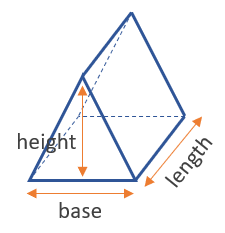# Volume of a Prism Calculator

Use this triangular prism calculator to easily calculate the volume of a triangular prism or tank from its length, base, and height in any metric: mm, cm, meters, km, inches, feet, yards, miles...

### Calculation results

30 cm3 Prism volume
Share calculator:

Embed this tool:
get code

## Volume of a triangular prism formula

The volume formula for a triangular prism is (height x base x length) / 2, as seen in the figure below:So, you need to know just three measures: height, base, and length, in order to calculate the volume.

## How to calculate the volume of a triangular prism?

You need to take or know (from a plan/schematic) three length measurements. Make sure they are all in the same length unit, or convert accordingly until they are. The resulting output from our triangular prism calculator is always in cubic units: in3, ft3, yd3, mm3, cm3, meters3, etc.

The math is fairly simple, so it can be done using an ordinary calculator as well as by hand, but it can be difficult with large numbers or numbers with fractions.

## Example: find the volume of a prism

Three measurements of a prism need to be known before the volume can be calculated using the equation above: the prism length, height, and base. For example, if the height is 5 inches, the base 2 inches and the length 10 inches, what is the prism volume? To get the answer, multiply 5 x 2 x 10 and divide the result by 2, getting 10 x 10 / 2 = 100 / 2 = 50 cubic inches.

## Practical applications

A lot of classical roofs have the shape of a triangular prism, so calculating the volume of air below it might be useful if you are using the space as a living area. This type of roof has the best distribution of forces generated by the weight of the roofing and lateral forces (i.e. wind). Many camping tents are also such prisms, making use of the same beneficial properties.

A triangular prism volume calculation may also be handy if you want to estimate the volume of a toblerone bar. One way to approach this curious problem is to first use the volume of a prism calculator above to calculate the volume of the bar, including the indentations. Then use it to estimate the volume lost to one indentation and multiply it by their number to get the actual chocolate filled volume.

#### Cite this calculator & page

If you'd like to cite this online calculator resource and information as provided on the page, you can use the following citation:
Georgiev G.Z., "Volume of a Triangular Prism Calculator", [online] Available at: https://www.gigacalculator.com/calculators/volume-of-triangular-prism-calculator.php URL [Accessed Date: 01 Apr, 2023].